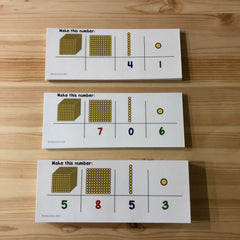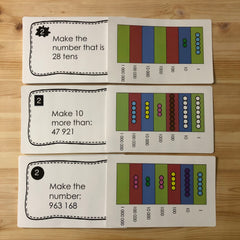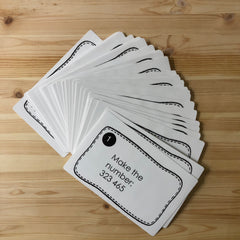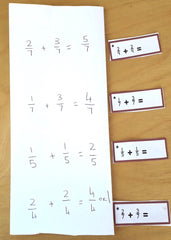# Montessori Math Bundle

Save \$84.90
\$424.48
\$339.58Montessori's tools helped bring abstract concepts into the concrete. This means that through hands on experience and manipulation children can learn complex ideas.

I have made a bundle of resources for you to download that will ease your montessori math journey. This is delivered as a digital resource to your inbox. No physical copy will be sent.

Montessori herself said:

The mathematical mind is a mind which is especially interested in mathematics. Instead of finding mathematics idiotic and absurd, it finds them interesting and absorbing. It is the fact that most of the children in our Montessori Schools do achieve great enthusiasm in doing mathematics. It is the preparation of their minds which enables them to derive this pleasure. --1946 London Lectures

This is why I have created my Math Bundle which has lots of premade materials that I have made through my time teaching. They are ready to print and go. Saving you valuable time and enabling you to meet the needs of those in front of you.

At 60+ items and 700+ pages there is loads in here for you at just a few cents per page. you can have a virtual basket full of tools you can dip into to help with many Montessori lessons.

See how all these resources link to the Montessori Math Scope and Sequence here. A free copy of the scope and sequence will also be in the bundle!

COUNTINGBefore and after to 10 (what number comes before and what comes after)

Clip cards for 1-20

Teen Numbers pack

DECIMAL SYSTEMIntroduction to Base 10

Basic Place value task cards (2 digit numbers)

Greater than and less than task cards

Make me game (make digits between 2 and 4 digits long)

OPERATIONS3 digit equations

4 digit equations

Checkerboard stories

Multiplication questions with 2 and 3 digit multipliers

BASIC FACTSDoubles (helps students see how knowing things like 4+ 4 or 6+ 6 can help them learn other basic facts)

Near Doubles (learning how doubles can help learn near doubles like 6 + 7)

Number bonds to 10 (using colored beads to help learn basic facts)

Number bonds to 18 (using colored beads to help learn basic facts)

Addition, Subtraction, Multiplication and Division Booklets

Fact families for add/sub (helps students see how inverse operations relate to each other)

Arrays (help children see the connection between addition and multiplication)

Pythagoras Board Task cards (for extending knowledge of multiplication facts)

Different formats for writing equations (Addition, Subtraction, Multiplication and Division)

FRACTIONS AND DECIMALSFraction Posters

Fraction circle clip cards

Fraction identification and sorting activity

Ordering fractions with the same denominator cards

Addition and subtraction of fraction tickets

Fraction chart to find fractions of an amount

Fractions of an amount activity

Addition and subtraction of decimals equations

Multiplying decimals by 10, 100 and 1000 task cards

Multiplying decimals by a whole number task cards

Ordering decimals on a number line clip and flip activity

Fraction, decimal, percentage flip book

Comparing decimals to fractions match up

Exploring fractions to decimals task cards

Exploring fractions to percentages task cards

STRUCTURE AND ALGEBRA

Gateway to Algebra questions 3 digit and 4 digit numbers

Factors worksheet

OTHER

Measurement activities

Probability activities

Graphing activities

Money activities

All current and future Montessori math resources for preschool and elementary students at one price. Including activities for colored beads, place value and extension.

785 pages

### You recently viewed

Clear recently viewed